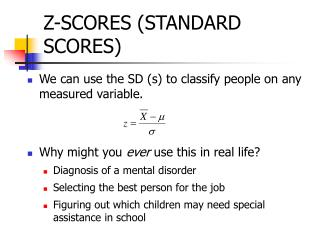DownloadDownload PresentationZ-SCORES (STANDARD SCORES)

# Z-SCORES (STANDARD SCORES)

Télécharger la présentation## Z-SCORES (STANDARD SCORES)

- - - - - - - - - - - - - - - - - - - - - - - - - - - E N D - - - - - - - - - - - - - - - - - - - - - - - - - - -
##### Presentation Transcript

1. Z-SCORES (STANDARD SCORES) • We can use the SD (s) to classify people on any measured variable. • Why might you ever use this in real life? • Diagnosis of a mental disorder • Selecting the best person for the job • Figuring out which children may need special assistance in school

2. EXAMPLE FROM I/O • Extraversion predicts managerial performance. • The more extraverted you are, the better a manager you will be (with everything else held constant, of course).

3. Scores for current managers 10, 25, 32, 35, 39, 40, 41, 45, 48, 55, 70 N=11 Need the mean Need the standard deviation AN EXTRAVERSION TEST TO EMPLOYEES

4. Let’s Do It

5. SOMEBODY APPLIES FOR A JOB AS A MANAGER • Obtains a score of 42. • Should I hire him? • Somebody else comes in and has a score of 44? What about her? • What if the mean were still 40, but the s = 2?

6. HARDER EXAMPLE: • Two people applying to graduate school • Bob, GPA = 3.2 at Northwestern Michigan • Mary, GPA = 3.2 at Southern Michigan • Whom do we accept? • What else do we need to know to determine who gets in?

7. SCHOOL PARAMETERS • NWMU mean GPA = 3.0; SD = .1 • SMU mean GPA = 3.6; SD = .2 • THE MORAL OF THE STORY: We can compare people across ANY two tests just by saying how many SD’s they are from the mean.

8. ONLY ONE TEST • it might make sense to “rescore” everyone on that test in terms of how many standard deviations each person is from the mean. • The “curve”

9. z-SCORES & LOCATION IN A DISTRIBUTION • Standardization or Putting scores on a test into a form that you can use to compare across tests. These scores become known as “standardized” scores. • The purpose of z-scores, or standard scores, is to identify and describe the exact location of every score in a distribution • z-score is the number of standard deviations a particular score is from the mean.(This is exactly what we’ve been doing for the last however many minutes!)

10. z-SCORES • The sign tells whether the score is located above (+) or below (-) the mean • The number (magnitude) tells the distance between the score and the mean in terms of number of standard deviations

11. WHAT ELSE CAN WE DO WITH z-SCORES? • Converting z-scores to X values • Go backwards. Aaron says he had a z-score of 2.2 on the Math SAT. • Math SAT has a m = 500 and s = 100 • What was his SAT score?

12. USING Z-SCORES TO STANDARDIZE A DISTRIBUTION • Shape doesn’t change (Think of it as re-labeling) • Mean is always 0 • SD is always 1 • Why is the fact that the mean is 0 and the SD is 1 useful? • standardized distribution is composed of scores that have been transformed to create predetermined values for m and s • Standardized distributions are used to make dissimilar distributions comparable

13. DEMONSTRATION OF A z-SCORE TRANSFORMATION • here’s an example of this in your book (on pg. 161). I’m not going to ask you to do this on an exam, but I do want you to look at this example. I think it helps to re-emphasize the important characteristics of z-scores.· The two distributions have exactly the same shape· After the transformation to z-scores, the mean of the distribution becomes 0· After the transformation, the SD becomes 1· For a z-score distribution, Sz = 0· For a z-score distribution, Sz2 = SS = N (I will not emphasize this point)

14. FINAL CHALLENGE • Using z-scores to make comparisons (Example from pg. 112) • Bob has a raw score of 60 on his psych exam and a raw score of 56 on his biology exam. • In order to compare, need the mean & the SD of each distribution • Psych: m = 50 and s=10 • Bio: m = 48 and s=4

15. FINAL CHALLENGE II • You could • sketch the two distributions and locate his score in each distribution • Standardize the distributions by converting every score into a z-score • OR • Transform the two scores of interest into z-scores • PSYCH SCORE = (60-50)/10 = 10/10 = +1 • BIO SCORE = (56-48)/4 = 8/4 = +2 • *Important element of this is INTERPRETATION*

16. OTHER LINEAR TRANSFORMATIONS • Steps for converting scores to another test • Take the original score and make it a z-score using the first test’s parameters • Take the z-score and turn it into a “raw” score using the second test’s parameters. • Standard Score = mnew + zsnew • See “Learning Checks” in text, these are a general idea of what might be on the exam